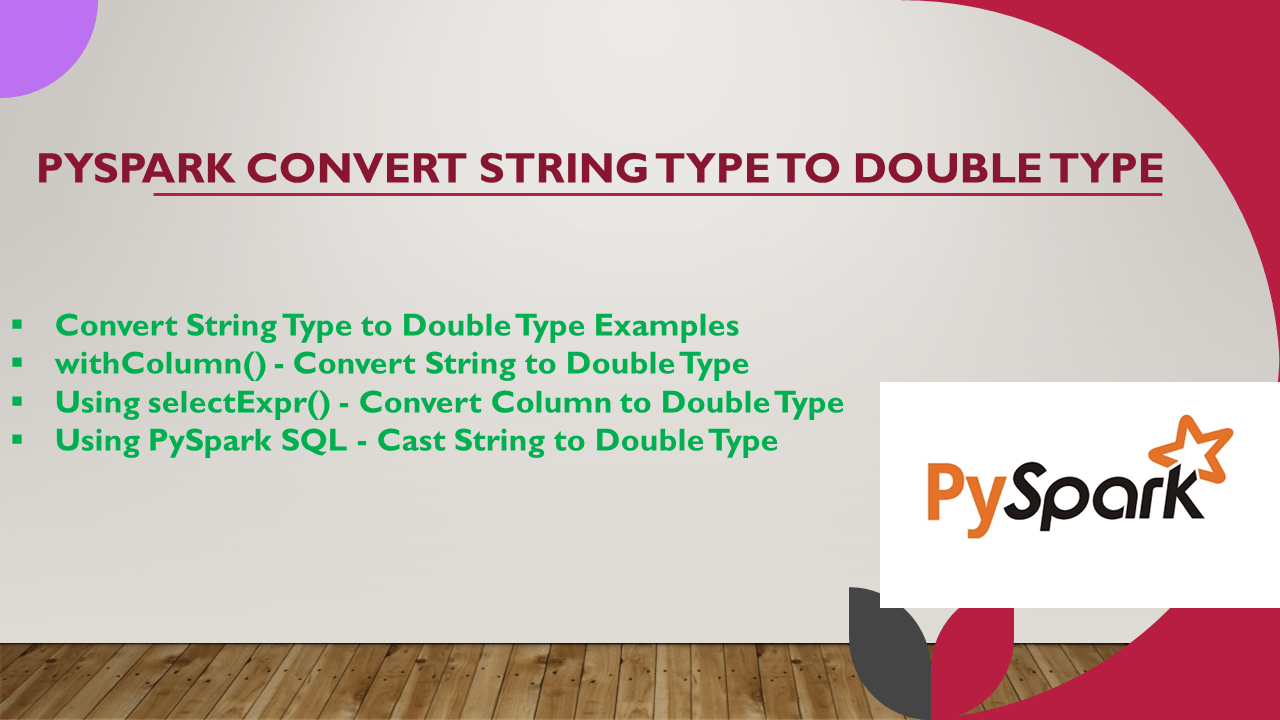# PySpark Convert String Type to Double Type

In PySpark SQL, using the `cast()` function you can convert the DataFrame column from String Type to Double Type or Float Type. This function takes the argument string representing the type you wanted to convert or any type that is a subclass of DataType.

Key points

• `cast()``cast()` is a function from `Column` class that is used to convert the column into the other datatype.
• This function takes the argument string representing the type you wanted to convert or any type that is a subclass of DataType.
• When Spark unable to convert into a specific type, it returns a null value.
• PySpark SQL takes the different syntax `DOUBLE(String column)` to cast types.

## 1. Convert String Type to Double Type Examples

Following are some PySpark examples that convert String Type to Double Type, In case if you wanted to convert to Float Type just replace the Double with Float.

``````
#Using withColumn() examples
df.withColumn("salary",df.salary.cast('double'))
df.withColumn("salary",df.salary.cast(DoubleType()))
df.withColumn("salary",col("salary").cast('double'))

# Rounds it to 2 digits
df.withColumn("salary",round(df.salary.cast(DoubleType()),2))

# Using select
df.select("firstname",col("salary").cast('double').alias("salary"))

# Using select expression
df.selectExpr("firstname","cast(salary as double) salary")

# using SQL to Cast
spark.sql("SELECT firstname,DOUBLE(salary) as salary from CastExample")
``````

Let’s run with some examples.

``````
from pyspark.sql import SparkSession
# Create SparkSession
spark = SparkSession.builder \
.appName('SparkByExamples.com') \
.getOrCreate()

simpleData = [("James",34,"true","M","3000.6089"),
("Michael",33,"true","F","3300.8067"),
("Robert",37,"false","M","5000.5034")
]

df = spark.createDataFrame(data = simpleData, schema = columns)
df.printSchema()
``````

Outputs below schema. Note that column `salary` is a string type.

## 2. withColumn() – Convert String to Double Type

First will use PySpark DataFrame withColumn() to convert the `salary` column from String Type to Double Type, this withColumn() transformation takes the column name you wanted to convert as a first argument and for the second argument you need to apply the casting method `cast()`.

``````
from pyspark.sql.types import DoubleType
from pyspark.sql.functions import col
df2 = df.withColumn("salary",df.salary.cast('double'))
#or
df2 = df.withColumn("salary",df.salary.cast(DoubleType()))
df2.printSchema()
``````

Outputs below schema & DataFrame

In case if you wanted round the decimal value, use the `round()` function.

``````
from pyspark.sql.types import DoubleType
from pyspark.sql.functions import col, round
df.withColumn("salary",round(df.salary.cast(DoubleType()),2))
.show(truncate=False)

# This outputs
+---------+---+-----------+------+-------+
+---------+---+-----------+------+-------+
|James    |34 |true       |M     |3000.61|
|Michael  |33 |true       |F     |3300.81|
|Robert   |37 |false      |M     |5000.5 |
+---------+---+-----------+------+-------+
``````

## 3. Using selectExpr() – Convert Column to Double Type

Following example uses selectExpr() transformation of SataFrame on order to change the data type.

``````
df3 = df.selectExpr("firstname","age","isGraduated","cast(salary as double) salary")
``````

## 4. Using PySpark SQL – Cast String to Double Type

In SQL expression, provides data type functions for casting and we can’t use `cast()` function. Below `DOUBLE(column name)` is used to convert to Double Type.

``````
df.createOrReplaceTempView("CastExample")
df4=spark.sql("SELECT firstname,age,isGraduated,DOUBLE(salary) as salary from CastExample")
``````

## 5. Conclusion

In this simple PySpark article, I have provided different ways to convert the DataFrame column from String Type to Double Type. you can use a similar approach to convert to Float types.

Happy Learning !!

### Naveen (NNK)

Naveen (NNK) is a Data Engineer with 20+ years of experience in transforming data into actionable insights. Over the years, He has honed his expertise in designing, implementing, and maintaining data pipelines with frameworks like Apache Spark, PySpark, Pandas, R, Hive and Machine Learning. Naveen journey in the field of data engineering has been a continuous learning, innovation, and a strong commitment to data integrity. In this blog, he shares his experiences with the data as he come across. Follow Naveen @ @ LinkedIn